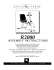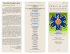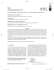Transcription

Pre-Calculus: Chapter 4 Review
Name: ______________________
This are problems that supplement the textbook review: pg.439: 1,5,13,19, 23, 29, 33, 35, 37, 41, 5155, 63. 75, 79,
81, -- 71, 85, 87, 97, 102
1. Find the exact values of the six trig functions of the angle Ѳ shown to
the right.
2. Find the five remaining trig functions of Ѳ, given
6
that sec  , tan   1
5
3. On graph paper, graph the function for two full cycles:
y  2 cos( x)  3
1  x
y   sin  
2 4
4. Find an equation for the
following graph.
5. Kylie measures the
angle of elevation from
a point on level ground
to the top of a building 120 meters high to be 32o . She walks towards the building until the angle of
elevation is 45o . How far does she walk?
6. Find all values of theta between 0    2 that satisfies the following equation. Do not use your
calculator for part a.
a. cot    3
b. sec  1.743
7. The following graph shows the depth of water, y
metres, at a point P, during one day.
The time t is given in hours, from midnight to noon.
(a)
Use the graph to write down an estimate of
the value of t when
(i)
the depth of water is minimum;
(ii)
the depth of water is maximum;
(2)
(b)
The depth of water can be modelled by the
function y = A cos (B (t – 1)) + C.
(i)
Show that A = 8.
(ii)
Write down the value of C.
(iii)
Find the value of B.
(6)
(c)
A sailor knows that he cannot sail past P when the depth of the water is less than 12 m. Calculate the
values of t between which he cannot sail past P.
(2)
8. A Ferris wheel with centre O and a radius of 15 metres is represented in the diagram below. Initially seat A is at
ˆB = π .
ground level. The next seat is B, where AO
6
(a)
Find the length of the arc AB.
(b)
Find the area of the sector AOB.
(c)
The wheel turns clockwise through an angle of
2π
. Find the height of A above the ground.
3
The height, h metres, of seat C above the ground after t minutes, can be modelled by the function
π

h (t) = 15 − 15 cos  2t   .
4

(d)
π
.
4
(i)
Find the height of seat C when t =
(ii)
Find the initial height of seat C.
(iii)
Find the time at which seat C first reaches its highest point.

SC0TT`Personal Toilet Seat Cover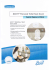BOAT SEAT - VA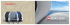ultimax.2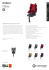Page 0458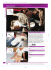Barcalounger Lectern II Recliner Chair Brochure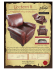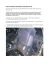2013 Class Dates - Safe Kids Grand Forks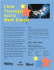recaro symbols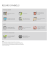Excellent Dining - Scandia Furniture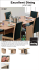February 8th at 12:45 pm CINEPLANET 16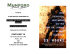Charlotte Ford relates her experience as a Barlow Robbins LLP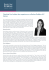How to transfer safely to the car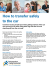Seat Return Guide - Fremantle Dockers Membership Home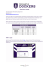Forward-facing Car Seats - Infant Toddler Safety Association..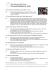How Much Can You Fit In Your Car?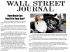Transporting children safely - Early Learning Association Australia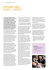to a pamphlet on multi-stage seats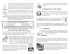Assembly Guide